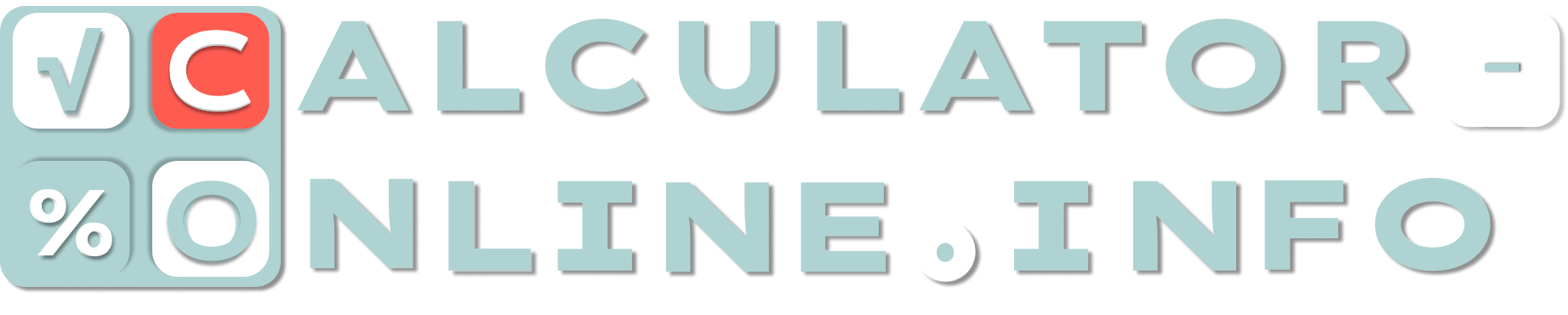# SLG Calculator & Formula. How is slugging percentage calculated?

This slugging percentage calculator is a useful tool for evaluating a baseball player’s performance. Knowing baseball statistics from previous competitions, you can easily determine how valuable a player is, because it will calculate the batting effectiveness of a hitter.

1st Base
2nd Bases
3rd Bases
Home runs
At-Bats

## What is slugging percentage?

What does slugging percentage mean in baseball? Slugging percentage is a kind of measure which stands for the power of a hitter. In some sense it is an average score of bases reached per bat. The higher the player’s score, the more likely the whole team wins.

The SLG abbreviation may also occur in baseball. It means the same (slugging percentage).

## How is slugging percentage calculated?

Baseball slugging percentage is based on the previous baseball statistics. To calculate slugging percentage it is necessary to divide total bases (the number of bases a player has gained) by the number of taken bats (turns of batter batting against a pitcher).

## How to calculate slugging percentage?

You need correct slugging percentage formula, which takes into account not only the number of bases a player has gained, but also a type of it. According to it each base has its own coefficient:

• 1× for the first base the player has reached;
• 2× for the second base;
• 3× for the third base;
• 4× for home run.

Thus, slugging percentage calculations are done by the following formula:

### SLG = (1st B + 2×2d B + 3×3d B + 4×HR) / AB

SLG is slugging percentage you are calculating.

1st B is a number of the first bases the player has reached.

2d B is a number of the second bases the player has reached.

3d B is a number of the third bases the player has reached.

HR is a number of home bases.

AB stands for “at-bats” and means a number of batting turns.

## What is a good slugging percentage?

All depends on the type of hitter. For example, there are always more requirements for a power hitter than for a contact hitter.

On average, it is considered that a good slugging percentage is 0.450 or higher. 0.550 is an outstanding score, while 0.650 and above would be one of the highest. If SLG is 0.350 or less it is called as fairly poor result.

## How do you calculate slugging percentage?

You can try to calculate the percentage using a formula or just enter the values ​​in SLG calculator fields and it will calculate slugging percentage by itself.

For example, a player has been at bat 45 times. Let’s imagine that he or she has reached the first base 5 times, the second base 4 times, the third base once and 2 home runs. In this case slugging calculator will help to determine the result in such way:

### SLG = (5 + 2×4 + 3×1 + 4×2) / 45

Total bases earned here is 24 (5 + 8 + 3 + 8), so it must be divided by 45 at bats. We will get SLG equal to 0.533 that is quite good result for a hitter.

Use our baseball calculator for SLG calculations to simplify and speed up the process.

Rating
( 3 assessment, average 5 from 5 )Mathematician/ author of the article